Anúncio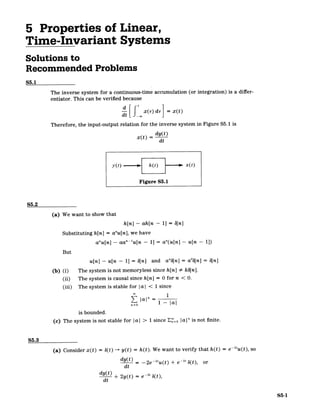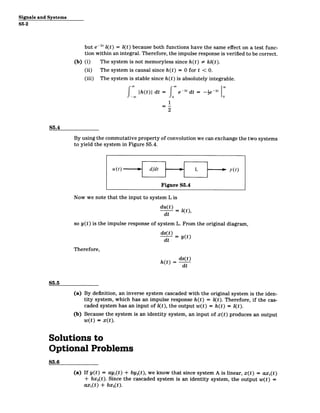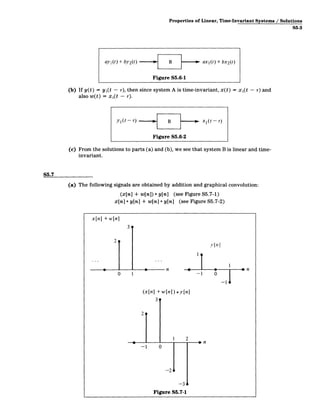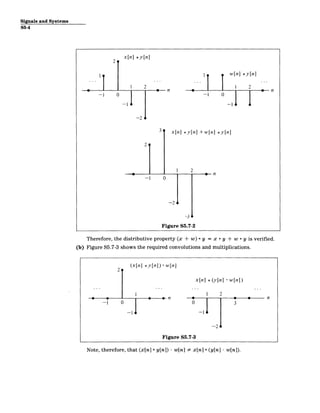Anúncio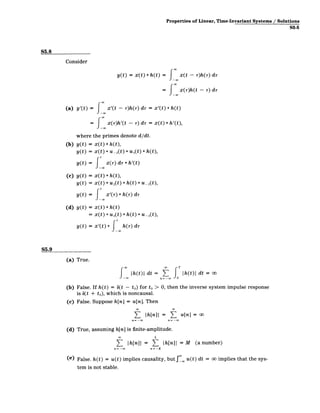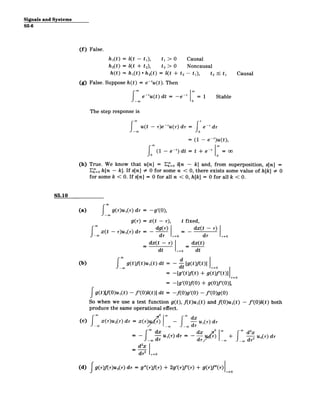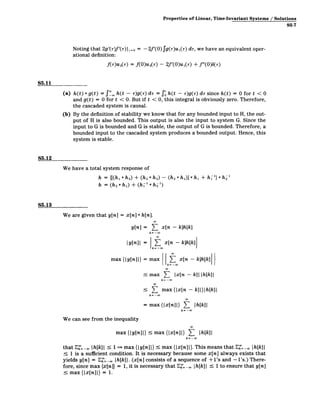Próximos SlideSharesDigital Signal Processing Assignment Help
Carregando em ... 3
1 de 8
Anúncio

### Assignment properties of linear time-invariant systems

1. 5 Properties of Linear, Time-Invariant Systems Solutions to Recommended Problems S5.1 The inverse system for a continuous-time accumulation (or integration) is a differ­ entiator. This can be verified because d[ x(r) dr =x(t) Therefore, the input-output relation for the inverse system in Figure S5.1 is dy(t) x(t) = dt y(t F h(t) x x(t) Figure S5.1 S5.2 (a) We want to show that h[n] - ah[n - 11 = b[n] Substituting h[n] = anu[n],we have anu[n] - aa"-u[n - 1] = a"(u[n] - u[n - 1]) But u[n] - u[n - 1] = b[n] and an6[n] = ab[n] = b[n] (b) (i) The system is not memoryless since h[n] # kb[n]. (ii) The system is causal since h[n] = 0 for n < 0. (iii) The system is stable for Ia I < 1 since 1 |al" 1 - |a is bounded. (c) The system is not stable for |al > 1 since E"o 1 a|" is not finite. S5.3 (a) Consider x(t) = 6(t) -+ y(t) = h(t). We want to verify that h(t) = e-2u(t), so dy(t) - -2e- 2 u(t) + e -' 6(t), or dt dy(t) + 2y(t) -21 6(t), =e dt S5-1
2. Signals and Systems S5-2 but ed' b(t) = 6(t) because both functions have the same effect on a test func­ tion within an integral. Therefore, the impulse response is verified to be correct. (b) (i) The system is not memoryless since h(t) # kb(t). (ii) The system is causal since h(t) = 0 for t < 0. (iii) The system is stable since h(t) is absolutely integrable. IhtI dt = e 2 t dt = -le -2t 2 S5.4 By using the commutative property of convolution we can exchange the two systems to yield the system in Figure S5.4. u(t) - d/dt L y(t) Figure S5.4 Now we note that the input to system L is du(t) = 6(t ), dt so y(t) is the impulse response of system L. From the original diagram, ds(t) dt = yt) Therefore, h(t) = ds(t) dt S5.5 (a) By definition, an inverse system cascaded with the original system is the iden­ tity system, which has an impulse response h(t) = 6(t). Therefore, if the cas­ caded system has an input of b(t), the output w(t) = h(t) = 6(t). (b) Because the system is an identity system, an input of x(t) produces an output w(t) = x(t). Solutions to Optional Problems S5.6 (a) If y(t) = ayi(t) + by2(t), we know that since system A is linear, x(t) = ax,(t) + bx 2 (t). Since the cascaded system is an identity system, the output w(t) = ax1(t) + bx 2(t).
3. Properties of Linear, Time-Invariant Systems / Solutions S5-3 ay1(t + by 2 (t) B axI(t) + bx2(t) Figure S5.6-1 (b) If y(t) = y1(t - r), then since system A is time-invariant, x(t) = x,(t - -) and also w(t) = xi(t - r). yI(t -r) B - xI(t- r) Figure S5.6-2 (c) From the solutions to parts (a) and (b), we see that system B is linear and time- invariant. S5.7 (a) The following signals are obtained by addition and graphical convolution: (x[n] + w[n]) * y[n] (see Figure S5.7-1) x[n] * y[n] + w[n] * y[n] (see Figure S5.7-2) x[n] +w[n] yInI 1? - 0 1 0 n - 1 6 -1 (x[n] +w[n]) *y[n] 39 1 2 -l 0 -3 6 Figure S5.7-1
4. Signals and Systems S5-4 x[n] *y[n] 2 2 1 0 n -0 1 9 n -1 0 -1 0 -2 3 x[n] *y[n] +w[n] *y[n] 2 1 2 -I 0 -2< -3 Figure S5.7-2 Therefore, the distributive property (x + w) * y = x * y + w * y is verified. (b) Figure S5.7-3 shows the required convolutions and multiplications. 2 (x[n] *y[n])-w[n] x[n] *(y[n] -w[n]) 1 1 2 n 1 0 n -1 0 0 3 -1 -1 -2 Figure S5.7-3 Note, therefore, that (x[n] * y[n]) - w[n] # x~n] * (y[n] - w[n]).
5. Properties of Linear, Time-Invariant Systems / Solutions S5-5 S5.8 Consider y(t) = x(t) * h(t) = J- x(t - r)h(r)dr = x(r)h(t - r) dr (a) y'(t) = x'(t - r)h(r) d-r = x'(t) * h(t) = x(r)h'(t - r) dr = x(t) * h'(t), where the primes denote d/dt. (b) y(t) = x(t) * h(t), y(t) = x(t) * u 1 (t) * ui(t) * h(t), y(t) = x(r) dr * h'(t) (c) y(t) = x(t) * h(t), y(t) = x(t) * ui(t) * h(t) * u _ 1(t), y(t) = x'(r) * h(r) dr (d) y(t) = x(t) *h(t) = x(t) * u(t) * h(t) * n 1(t), y(t) = Xt) * h(r) dr S5.9 (a) True. f0 |h(t)| dt = fT |h(t)| dt = oo (b) False. If h(t) = 3(t - to) for to > 0, then the inverse system impulse response is b(t + to), which is noncausal. (c) False. Suppose h[n] = u[n]. Then Z Ih[n]| = [ u[n] = c n= -oo n= -oo (d) True, assuming h[n] is finite-amplitude. 0o L Zh[n]I = E Ih[n] = M (a number) n=-co n=-K (e) False. h(t) = u(t) implies causality, but J u(t) dt = oo implies that the sys­ tem is not stable.
6. Signals and Systems S5-6 (f) False. hi(t) = 6(t - ti), ti > 0 Causal h 2(t) = b(t + 2 ), t2 > 0 Noncausal h(t) = hI(t) * h2(t) = 6(t + t 2 - ti), t 2 !- t 1 Causal (g) False. Suppose h(t) = e-'u(t). Then e- tu(t)dt = -e-' -1 Stable 0 The step response is u(t - r)e- T u(r) d-r dr e -T = ( (1 - e~')u(t), (1 - e -') dt =t + e-'1 = oo (h) True. We know that u[n] = E=O b[n - k] and, from superposition, s[n] = Ef=0 h[n - k]. If s[n] # 0 for some n < 0, there exists some value of h[k] # 0 for some k < 0. If s[n] = 0 for all n < 0, h[k] = 0 for all k < 0. S5.10 (a) J g(r)u1(r) dr = -g'(0), g(r) = x(t - r), t fixed, - x(t - )u1(r) dr= dg(r) _ _ dx(t - -r) Jodr r=o dr t=0 dx(t - r) dx(t) dt r=O dt (b) g(t)f(t)uj(t) dt - dt [gt)ft)]_ = = -[g'(t)ftt) ± g(t)f'(t)]__ - IgMAO+ Xt 1(l It =0 = -[g'(O)f(O) + g(0)f'(0)], Tg(t)[f(O)ui(t) - f'(0)S(t)] dt = -f(0)g'(0) - f'(0)g(0) So when we use a test function g(t), f(t)ui(t) and f(O)ui(t) - f'(0)6(t) both produce the same operational effect. (c) x(r)u2(r) dr = x(r)) - f -u (r)dr -- o _Y6 _.dr *~dx dx/ d2X -J -uir) dr = 7 ) + d2U0(r) dr d2 x dr2 r=0 (d) fg(r)fr)u2(r) dr = g"(r)f(r) + 2g'(r)f'(r) + g(r)f"(r)
7. Properties of Linear, Time-Invariant Systems / Solutions S5-7 Noting that 2g'(7r)f'(r) ,= = - 2f'(O) fg(r)ui(r)dr, we have an equivalent oper­ ational definition: f(r)u 2(r) = f(O)u 2(r) - 2f'(0)ui(r) + f"(O)b(r) S5.11 (a) h(t) * g(t) = J'. h(t - r)g(r) dr = f' h(t - )g(r) dr since h(t) = 0 for t < 0 and g(t) = 0 for t < 0. But if t < 0, this integral is obviously zero. Therefore, the cascaded system is causal. (b) By the definition of stability we know that for any bounded input to H, the out­ put of H is also bounded. This output is also the input to system G. Since the input to Gis bounded and G is stable, the output of Gis bounded. Therefore, a bounded input to the cascaded system produces a bounded output. Hence, this system is stable. S5.12 We have a total system response of h = {[(h, * h 2 ) + (h 2 * h 2 ) - (h 2 * hl)] * hi + h-i} * hl h = (h 2 * h) + (hi 1 * h 2 ') S5.13 We are given that y[n] = x[n] * h[n]. y[n] = ( x[n - k]h[k] k= -w Iy[n]I = E x[n - k]h[k] k=-Oo max{y[n]|} = max x[n - k]h[k] k=-o < E max {Ix[n- k]|}h[k]| k= -o =maxm{x n]l} z: lh[k]| k= -o We can see from the inequality max{Iy[n]|) max{|x[n]|} Z Ih[k]| k= -o0 that E Ih[k]| 5 1 max{Iy[n]|} s max {Ix[n]|}. This means that E Ih[k]I - 1 is a sufficient condition. It is necessary because some x[n] always exists that yields y[n] = E=_ Ih[k]1. (x[n] consists of a sequence of +1's and -l's.) There­ fore, since max {x[n]} = 1, it is necessary that E= h[k] 1 5 1 to ensure that y[n] S max {Ix[n]I} = 1.
8. MIT OpenCourseWare http://ocw.mit.edu Resource: Signals and Systems Professor Alan V. Oppenheim The following may not correspond to a particular course on MIT OpenCourseWare, but has been provided by the author as an individual learning resource. For information about citing these materials or our Terms of Use, visit: http://ocw.mit.edu/terms.
Anúncio